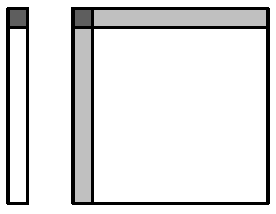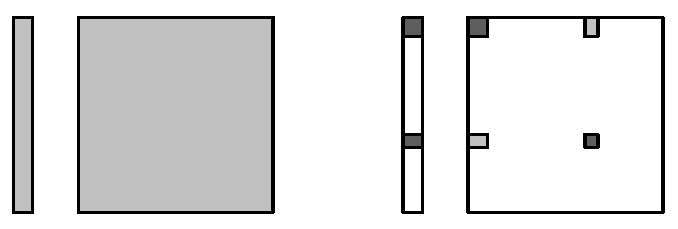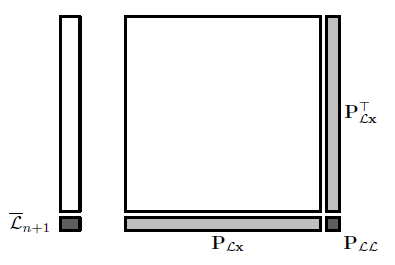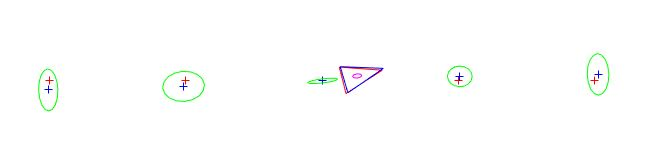# EKF-SLAM simulated in MATLAB

Reference:

Simulataneous localization and mapping with the extended Kalman filter - A very quick guide... with Matlab code!', Joan Sola, 5 Oct, 2014

# EKF预测环节# EKF更新环节

EKF中观测方程的形式为：

EKF中更新的形式为：

y是我们处理过的观测数据，比如路标是一个球，我们的维持的y是球心在图像平面的坐标，uv。

EKF中观测是一个一个处理的（应该有批量处理的办法？）K的复杂度是线性的O(n)。

## 全观测# 代码

    %II.1 SIMULATOR
%a.motion
n = q .* randn(2,1); %perturbation vector
R = move(R, U, zeros(2,1));

%b. observations
for i=1:N
v = s.* randn(2,1) % measurement noise
Y(:,i)=observe(R,W(:,i))+v; %add measurement noise to observations
end

%II.2 ESTIMATOR
%a. create dynamic map pointers to be used hereafter
m = landmarks(landmarks~=0)'; %all pointers to landmarks --- 指向状态量X的第某个元素
%[0 0 0 0 4 0 6 0; 0 0 0 0 5 0 7 0]表示5号路标对应X的第4，5个元素。
rm = [r, m]; %all used states: robot and landmarks ---rm=[123,67]
% ( also OK is rm = find(mapspace); )

% b. Prediction ---robot motion
[x(r), R_r, R_n] = move(x(r),U,n); %r=[1 2 3] %plus perturbation on estimation
P(r,m)=R_r * P(r,m);%updates the covariance matrix
P(m,r)=P(r,m)';
P(r,r)=R_r*P(r,r)*R_r'+R_n*Q*R_n';

%c. Landmark correction --- known landmarks
lids = find(landmarks(1,:)); %returns all indices of existing landmarks
for i = lids
%expectation: gaussian {e,E}
l = landmarks(:,i)'; %landmark pointer
[e,E_r,E_l]=observe(x(r),x(l));%this is h(x) in EKF
rl = [r,l]; %pointers to robot and lmk.
E_rl = [E_r, E_l]; % expectation Jacobian
E = E_rl * P(rl, rl) * E_rl';
% the block E is consist of robot pose 3 dimension and landmarker 2
% dimension, So it is 5*5 dimens

%measurement of landmark i
Yi =Y(:,i);
%innovation: Gaussian {z,Z}
z = Yi - e; %this is z = y - h(x) in EKF
%we need values around zero for angles:
if z(2)>pi
z(2)=z(2)-2*pi;
end
if z(2)<-pi
z(2)=z(2)+2*pi;
end
Z=S+E;

%Individual compatibility check at Mahalanobis distance of 3-sigma
% (See appendix of documentation file 'SLAM course.pdf')
if z'*Z^-1*z<9
%kalman gain
K=P(rm,rl)*E_rl'*Z^-1;%this is K = P*H'*Z^-1 in EKF
%map update (use pointer rm)
x(rm)=x(rm)+K*z;
P(rm,rm)=P(rm,rm)-K*Z*K';
end
end

%d. Landmark Initialization --- one new landmark only at each iteration
lids = find(landmarks(1,:)==0); % all non-initialzed landmarks
if ~isempty(lids) %there are still landmarks to initialize
i = lids(randi(numel(lids))); %pick one landmark randomly, its index is i
l = find(mapspace==false,2) %pointer of the new landmark in the map
%[1 1 1 1 1 0 0 ... 0 0]mapsapce，逻辑数组，表示对应位置的状态量X是否启用。
% l 返回6和7
if ~isempty(l)  %there is still sapce in the map
mapspace(l) = true; %block map space
landmarks(:,i)=l;%store landmark pointers
%measruement
Yi=Y(:,i);
%initialization
[x(l),L_r,L_y]=invObserve(x(r),Yi);
P(l,rm)=L_r*P(r,rm);%add P_lr P_rl P_ll to covariance matrix
P(rm,l)=P(l,rm)';
P(l,l)=L_r*P(r,r)*L_r'+L_y*S*L_y';
end
end

# 问题P(l,l)=L_r*P(r,r)*L_r'+L_y*S*L_y';

    L_r=[...
[1,0,-py*cos(a) - px*sin(a)]
[0,1,px*cos(a) - py*sin(a)]];

Reference:

Simulataneous localization and mapping with the extended Kalman filter - A very quick guide... with Matlab code!', Joan Sola, 5 Oct, 2014

03-05
05-0610-161万+
10-151万+
04-30
11-01
11-284871
11-301万+